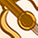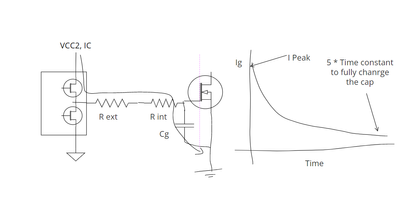Announcements

### Join the Asia Pacific Road to Decarbonization Seminar to get equipped as your embark on the journey into the future of sustainability.# 1EDI20N12AF Design QuestionLevel 3
Level 3Hello IFXer,

How to know the current required by the external converter of the 1EDI20N12AF according to the following condition?

Fs 60kHz

Src. / Sink current 4A/4A

VDD1 3.3V

VDD2 18V

MOSFET Qg 150nC

ex.

Pd = fs * Qg * V * Rg = 60kHz * 150nC * 18V * 3.3ohm = 0.55W

I = Pd / V = 0.55W / 18V = 30mA

1 Solution

# Re: 1EDI20N12AF Design QuestionModeratorModeratorHello,

You can use the below approach to calculate the current drawn from the secondary side. The current drawn from the secondary side is the sum of quiescent current and gate charging current. Quiescent current is already specified in the datasheet. Only gate charging current has to be calculated. Gate charging current is shown in fig. Calculate the average current from the below waveform.Please use our simulation models to calculate the current in different conditions. This provides more accurate results than the hand calculations, since this current depends on many factors like Temp, MOSFET, Rg etc.

Also adding the link for the gate resistor calculation for the power device.

https://www.infineon.com/dgdl/Infineon-EiceDRIVER-Gate_resistor_for_power_devices-ApplicationNotes-v...

Coming to your formula, there is an additional R in the Pd formula given by you. If you add an R, LHS unit and RHS unit will not match. Power dissipation should be Pd =  fs * Qg * V

Pd =  fs * Qg * V = (1/Second)*(Coulomb)*(Volt) = (Coulomb/Second)*Volt = Ampere * Volt = Watts

Power dissipation on the Rg_ext  and Rg int can be calculated as below.

Pd_Rg_ext = fs * Qg * V * ( Rg_ext /(Rg_ext + Rg_int))

Pd_Rg_int = fs * Qg * V * ( Rg_int /(Rg_ext + Rg_int))

Basically we are multiplying the voltage across the resistor and current.

To get the voltage across the resistor , we need to multiply the supply voltage against the voltage divider ratio made by R_ext and R_int.

Hope this explanation helps you,

If you have further questions, please let me know.

Thank you

Vishnu Nambrath

# Re: 1EDI20N12AF Design QuestionModeratorModeratorHello,

You can use the below approach to calculate the current drawn from the secondary side. The current drawn from the secondary side is the sum of quiescent current and gate charging current. Quiescent current is already specified in the datasheet. Only gate charging current has to be calculated. Gate charging current is shown in fig. Calculate the average current from the below waveform.Please use our simulation models to calculate the current in different conditions. This provides more accurate results than the hand calculations, since this current depends on many factors like Temp, MOSFET, Rg etc.

Also adding the link for the gate resistor calculation for the power device.

https://www.infineon.com/dgdl/Infineon-EiceDRIVER-Gate_resistor_for_power_devices-ApplicationNotes-v...

Coming to your formula, there is an additional R in the Pd formula given by you. If you add an R, LHS unit and RHS unit will not match. Power dissipation should be Pd =  fs * Qg * V

Pd =  fs * Qg * V = (1/Second)*(Coulomb)*(Volt) = (Coulomb/Second)*Volt = Ampere * Volt = Watts

Power dissipation on the Rg_ext  and Rg int can be calculated as below.

Pd_Rg_ext = fs * Qg * V * ( Rg_ext /(Rg_ext + Rg_int))

Pd_Rg_int = fs * Qg * V * ( Rg_int /(Rg_ext + Rg_int))

Basically we are multiplying the voltage across the resistor and current.

To get the voltage across the resistor , we need to multiply the supply voltage against the voltage divider ratio made by R_ext and R_int.

Hope this explanation helps you,

If you have further questions, please let me know.

Thank you

Vishnu Nambrath